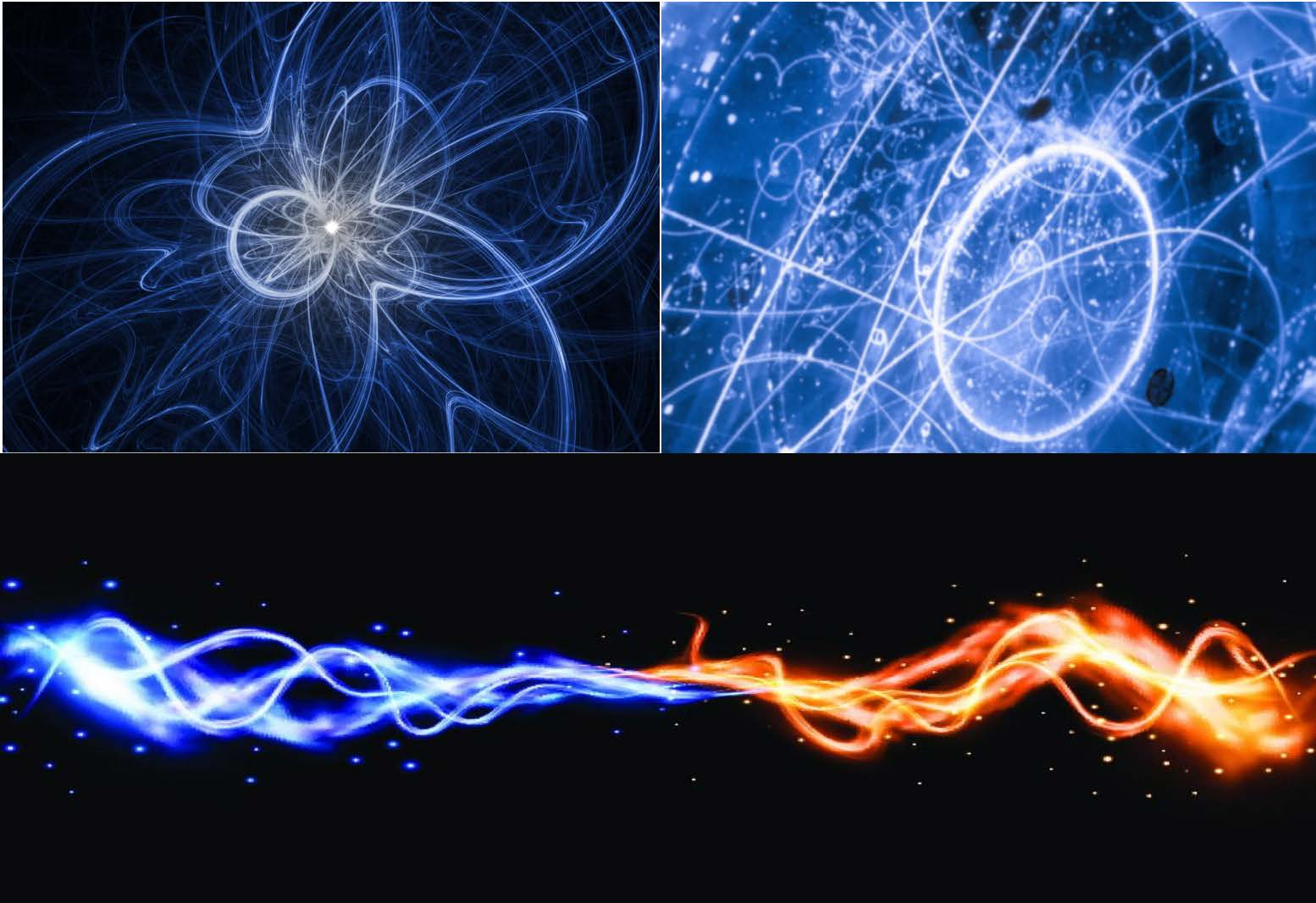Home  /  Kinetic theory: novel statistical, stochastic and analytical methods

# Program

Kinetic theory: Novel statistical, stochastic and analytical methods August 18, 2025 to December 19, 2025
Organizers Laurent Desvillettes (Université de Paris VII (Denis Diderot)), Irene M. Gamba (University of Texas, Austin), Francois Golze (École Polytechnique), LEAD Pierre Emmanuel Jabin (Pennsylvania State University), Qin Li (University of Wisconsin-Madison), Chiara Saffirio (Universität Basel), Lexing Ying (Stanford University)
DescriptionTop: Neutrino interactions and neutrino-atom interactions. Bottom: Collision of two "waves"
The focus of the proposed program is on so-called kinetic equations, describing the evolution of the of many-particle interacting systems. These models have the form of statistical flows, with their solutions being either a single or multiple point probability density functions or measures, supported in a space of attributes. The attributes are problem-dependent and can be molecular velocity, energy, opinion, wealth, and many others. The flow then predicts the evolution of the probability measure in time, position in space, and the interchanging of the particles' states by the transition probability. Probably the most classical kinetic equation is the Boltzmann equation which describes the evolutions of the phase-space density function for a dilute gas under binary molecular collisions. Other well-known classical kinetic models include the Landau equation, Vlasov equation for plasmas or other systems, Fokker-Planck equations or kinetic formulations of various macroscopic or hyperbolic systems. In recent years, the successes of kinetic theory gave rise to an rapidly increasing variety of mathematical models beyond physics to applications in life sciences, social sciences, economy. Even more recently fascinating connections between kinetic theory and some aspects of data science have emerged. Kinetic theory has strong and fascinating interactions with a large variety of other fields, including statistical mechanics, stochastic processes, dynamical systems... The program will strive to give an overview of the novel mathematical tools used in kinetic theory through a broad range of classical and more recent applications.
Keywords and Mathematics Subject Classification (MSC)
Tags/Keywords
• Kinetic theory

• collisional models

• instabilities

• turbulence

• many-particle and multi-agent systems

• stochastic systems

• mean-field games

• mean-field equations

Primary Mathematics Subject Classification
Secondary Mathematics Subject Classification No Secondary AMS MSC
Logistics Program Logistics can be viewed by Members. If you are a program member then Login Here.
Programmatic Workshops Workshop dates have not yet been selected